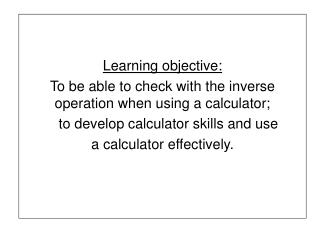DownloadDownload PresentationLearning objective: To be able to check with the inverse operation when using a calculator; to develop calculator sk

# Learning objective: To be able to check with the inverse operation when using a calculator; to develop calculator sk

Download Presentation## Learning objective: To be able to check with the inverse operation when using a calculator; to develop calculator sk

- - - - - - - - - - - - - - - - - - - - - - - - - - - E N D - - - - - - - - - - - - - - - - - - - - - - - - - - -
##### Presentation Transcript

1. Learning objective: To be able to check with the inverse operation when using a calculator; to develop calculator skills and use a calculator effectively.

2. Using a calculator work out and record in your books the answers to these: 21247 + 43578 56372 - 8657 387 x 257 5952 ÷ 372 Q. How could you use the calculator to check the answers?

3. You can check the answer by using the inverse operation. LOOK… 21247 + 43578 = 64825 64825 – 43578 = 21247 56372 - 8657 = 47715 47715 + 8657 = 56372 387 x 257 = 99459 99459 ÷ 387 = 257 5952 ÷ 372 = 16.01882 16.01882 x 372 = 5952

4. Check these with a partner on a calculator: 3496 x 27 = 90896 25398 + 76219 = 101617 467 x 286 = 133562 48602 ÷ 271 = 179.3432 63718 – 7534 = 56184 31263 ÷ 458 = 68.25983

5. Learning objective 2: To develop calculator skills and use a calculator effectively; to choose and use appropriate number skills to solve work problems; to check with the inverse operation when using a calculator.

6. Quiz to identify calculator keys and their functions

7. Key in£4.35 + £3.85 Do the sum You should see8.2 Q. What is the answer to the question? 8.2really means£8.20 How can we check this answer? What sum can we do using the inverse operation? Try it. We can do 8.2 - 4.35 or 8.2 - 3.85

8. Q. How would we enter £6.30? and 85p? Q. How would we enter six pounds and five pence? Is it £6.5 As we cannot used mixed units in the same calculation we must enter £6.30 + 85p as 6.30 + 0.85

9. Try these. Remember to check each answer using the inverse calculation.

10. Volunteers needed… to explain what they did when their checking showed a wrong answer. Q. Which was the trickiest question? Why?

11. LOOK… £3.95 + 75p + 95p = £173.95 Q. Can this answer be right? What might have gone wrong?

12. By the end of this lesson the children should be able to: Clear the display before entering a calculation; use the [+];[-]; [x] and [÷] key and decimal point to calculate; change an accidental wrong entry by using the [clear entry] key; key in and interpret money calculations; have a feel for the approximate size of an answer and check it by performing the inverse calculation.